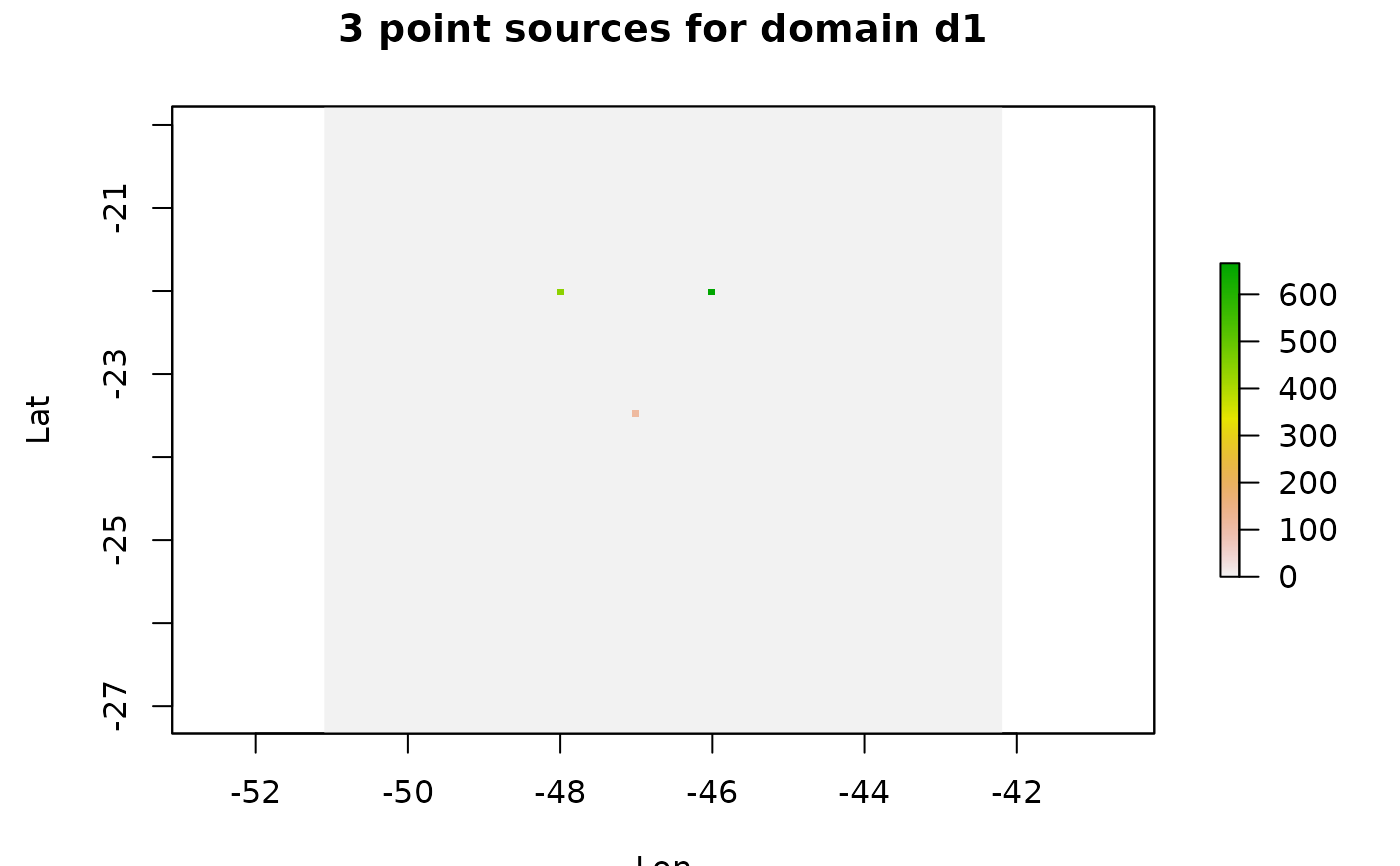Transform a set of points into a grinded output

pointSource(emissions, grid, verbose = T)

## Arguments

emissions list of points grid object with the grid information display additional information

## Value

a raster

gridInfo and rasterSource

## Examples

d1 <- gridInfo(paste(system.file("extdata", package = "EmissV"),"/wrfinput_d01",sep=""))#> Grid information from: C:/Users/Schuch/Documents/EmissV/inst/extdata/wrfinput_d01
p = data.frame(lat      = c(-22,-22,-23.5),
lon      = c(-46,-48,-47  ),
z        = c(0  ,  0,  0  ),
emission = c(666,444,111  ) )

p_emissions <- pointSource(emissions = p, grid = d1)#> grid position lat= -22.010806627171 lon= -46.0152584808041
#> grid position lat= -22.010806627171 lon= -47.9944115841027
#> grid position lat= -23.4723500487625 lon= -47.0048350324534 sp::spplot(p_emissions,scales = list(draw=TRUE), ylab="Lat", xlab="Lon",
main = "3 point sources for domain d1")